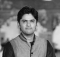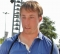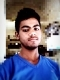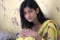## Difference Between Greedy Method and Dynamic ProgrammingUpdated on 02-Mar-2021 05:04:41
In this post, we will understand the differences between the greedy algorithm and dynamic programming methods.Greedy algorithmIt is an algorithmic paradigm that builds up on a solution in parts, step by step. The next step is chosen such that it gives the most obvious and immediate benefit.Problems that involve choosing local optimal values will help in choosing the global optimal values/solution to the problem. Such ate the problems associated with greedy algorithm.There is no surety that a greedy algorithm would lead to an optimal solution.An optimal choice is made at every stage of the problem, i.e the local optimal solution.It ... Read More

## Introduction to Greedy AlgorithmsUpdated on 30-Jul-2019 22:30:23
Greedy algorithm is designed to achieve optimum solution for a given problem. In greedy algorithm approach, decisions are made from the given solution domain. As being greedy, the closest solution that seems to provide an optimum solution is chosen. Greedy algorithms try to find a localized optimum solution, which may eventually lead to globally optimized solutions. However, generally greedy algorithms do not provide globally optimized solutions. In this Section We are going to cover − Activity Selection Problem Dijkstra’s Algorithm for Adjacency List Representation Dijkstra’s Shortest Path Algorithm Huffman Coding Algorithm Efficient Huffman Coding for Sorted Input Job Sequencing ... Read More

## Fractional Knapsack ProblemUpdated on 15-Jun-2020 17:07:11
A list of items is given, each item has its own value and weight. Items can be placed in a knapsack whose maximum weight limit is W. The problem is to find the weight that is less than or equal to W, and value is maximized.There are two types of Knapsack problem.0 – 1 KnapsackFractional KnapsackFor the 0 – 1 Knapsack, items cannot be divided into smaller pieces, and for fractional knapsack, items can be broken into smaller pieces.Here we will discuss the fractional knapsack problem.The time complexity of this algorithm is O(n Log n).Input and OutputInput: Maximum weight = ... Read More

## Prim’s MST for Adjacency List RepresentationUpdated on 15-Jun-2020 17:13:06
It is similar to the previous algorithm. Here the only difference is, the Graph G(V, E) is represented by an adjacency list.Time complexity adjacency list representation is O(E log V).Input and OutputInput: The cost matrix: Output: Edge: A--B And Cost: 1 Edge: B--E And Cost: 2 Edge: A--C And Cost: 3 Edge: A--D And Cost: 4 Edge: E--F And Cost: 2 Edge: F--G And Cost: 3 Total Cost: 15Algorithmprims(g: Graph, start)Input −  The graph g and the seed vertex named ‘start’Output − The Tree after adding edges.Begin    create two set B, N    add the start node in B ... Read More

## Prim’s Minimum Spanning Tree AlgorithmUpdated on 15-Jun-2020 17:22:04
There is a connected graph G(V, E) and the weight or cost for every edge is given. Prim’s Algorithm will find the minimum spanning tree from the graph G. It is a growing tree approach. This algorithm needs a seed value to start the tree. The seed vertex is grown to form the whole tree.The problem will be solved using two sets. One set holds the nodes that are already selected, and another set holds the item those are not considered yet. From the seed vertex, it takes adjacent vertices, based on minimum edge cost, thus it grows the tree by ... Read More

## Minimum Number of Platforms ProblemUpdated on 15-Jun-2020 15:48:57
A list of arrival and departure time is given. Now the problem is to find the minimum number of platforms are required for the railway as no train waits.By sorting all timings in sorted order, we can find the solution easily, it will be easy to track when the train has arrived but not left the station.The time complexity of this problem is O(n Log n).Input and OutputInput: Lists of arrival time and departure time. Arrival: {900, 940, 950, 1100, 1500, 1800} Departure: {910, 1200, 1120, 1130, 1900, 2000} Output: Minimum Number of Platforms Required: 3AlgorithmminPlatform(arrival, departure, int n)Input − The ... Read More

## Minimum Coin Change ProblemUpdated on 15-Jun-2020 15:51:47
There is a list of coin C(c1, c2, ……Cn) is given and a value V is also given. Now the problem is to use the minimum number of coins to make the chance V.Note − Assume there are an infinite number of coins CIn this problem, we will consider a set of different coins C{1, 2, 5, 10} are given, There is an infinite number of coins of each type. To make change the requested value we will try to take the minimum number of coins of any type.As an example, for value 22 − we will choose {10, 10, 2}, ... Read More

## Kruskal’s Minimum Spanning Tree AlgorithmUpdated on 15-Jun-2020 16:00:50
There is a connected graph G(V, E) and the weight or cost for every edge is given. Kruskal’s algorithm will find the minimum spanning tree using the graph and the cost.It is the merge-tree approach. Initially, there are different trees, this algorithm will merge them by taking those edges whose cost is minimum, and form a single tree.In this problem, all of the edges are listed and sorted based on their cost. From the list, edges with minimum costs are taken out and added in the tree, and every there is a check whether the edge forming cycle or not, ... Read More

## Job Sequencing Problem with DeadlinesUpdated on 15-Jun-2020 16:04:43
In this problem, there is a list of jobs given. In the list, the deadline and the profits are also given for each job. Every job will take a single unit of time, so the minimum deadline for a job is 1. If only one job can be scheduled at a time, then maximize the profit.To solve this problem, all subset of the set of jobs are generated to check whether the individual subset is feasible or not. Also, keep track on maximum profit for all feasible subset that has generated.The time complexity of this algorithm is O(n^2)Input and OutputInput: ... Read More

## Efficient Huffman Coding for Sorted InputUpdated on 15-Jun-2020 16:08:20
In the previous Huffman code problem, the frequency was not sorted. If the frequency list is given in sorted order, the task of assigning code is being more efficient.In this problem, we will use two empty queues. Then create a leaf node for each unique character and insert it into the queue in increasing order of frequency.In this approach, the complexity of the algorithm is O(n).Input and OutputInput: Different letters and their frequency in sorted order Letters: {L, K, X, C, E, B, A, F} Frequency: {1, 1, 2, 2, 2, 2, 3, 4} Output: Codes for the letters L: ... Read More